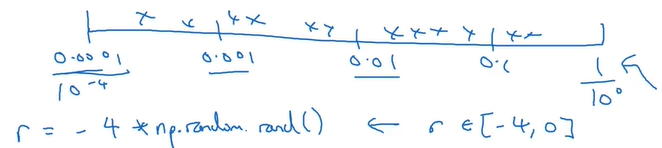# 一、超参数的取值

1. 首先大范围随机选择参数，根据随机取点测试超参数的效果，确定影响最大的参数是哪个。因为对于实际问题而言，很难确定哪个超参数对结果的影响更大，在众多参数中如果一个一个测试往往找不到需要调试的参数。
2. 采用由粗糙到精细的策略，找到随机测试中结果较好的参数范围，然后放大这一范围，在其中更密集的取值测试，搜索超参数的最优选择。# 二、Batch-Normalization(BN)

## 2.1 BN的基本思路

$\mu=\frac{1}{m}\sum_i z^{(i)}$
$\sigma^2=\frac{1}{m}\sum_i(z_i-\mu)^2$
$Z^{(i)}_{norm}=\frac{z^{(i)}-\mu}{\sqrt{\sigma^2+\epsilon}}$

$\widetilde z^{(i)}=\gamma z_{norm}^{(i)}+\beta$

## 2.2 BN为何有效

BN可以使权重比网络更滞后或更深层。

# 三、Softmax

## 3.1 softmax数学表示

$z^{[l]}=w^{[l]}a^{[l-1]}+b^{[l]}$

$t=e^{z^{[L]}}$

$a^{[l]}=\frac{t_i}{\sum t_i}$

## 3.2 训练softmax网络

（1）损失函数

$l(\hat y,y)=-\sum_{j=1}y_jlog\hat y_j$

（2）梯度下降

$dz^{[l]}=\hat y-y$